Skip to main content

Thank you for visiting nature.com. You are using a browser version with limited support for CSS. To obtain the best experience, we recommend you use a more up to date browser (or turn off compatibility mode in Internet Explorer). In the meantime, to ensure continued support, we are displaying the site without styles and JavaScript.

# Ergodic dynamics and thermalization in an isolated quantum system

## Abstract

Statistical mechanics is founded on the assumption that all accessible configurations of a system are equally likely. This requires dynamics that explore all states over time, known as ergodic dynamics. In isolated quantum systems, however, the occurrence of ergodic behaviour has remained an outstanding question1,2,3,4. Here, we demonstrate ergodic dynamics in a small quantum system consisting of only three superconducting qubits. The qubits undergo a sequence of rotations and interactions and we measure the evolution of the density matrix. Maps of the entanglement entropy show that the full system can act like a reservoir for individual qubits, increasing their entropy through entanglement. Surprisingly, these maps bear a strong resemblance to the phase space dynamics in the classical limit; classically, chaotic motion coincides with higher entanglement entropy. We further show that in regions of high entropy the full multi-qubit system undergoes ergodic dynamics. Our work illustrates how controllable quantum systems can investigate fundamental questions in non-equilibrium thermodynamics.

## Main

Imagine air molecules in a room. They move around with all possible velocities in all directions. Attaining the exact knowledge of these trajectories is a daunting and an unrealistic task. Statistical mechanics, however, claims that exact knowledge of individual trajectories is not required and systems can be accurately described using only a few parameters. What is the essential property of these systems that allows for such a simple description?

Ergodic dynamics provide an explanation for this simplicity. If the dynamics are ergodic, then the system will uniformly explore all microscopic states over time, constrained only by conservation laws. Ergodicity ensures that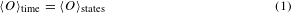where O is any macroscopic observable and brackets denote averaging. In the limit of many particles, macroscopic observables such as pressure or density approach equilibrium and are stationary—therefore, at any time, O(t) = 〈Otime. These two equations imply that macroscopic observables can be predicted by uniformly averaging over all states, and this forms the foundation for all thermodynamic calculations.

In classical systems, it is chaotic motion which drives the system to ergodically explore the state space5. Quantum systems, however, are governed by Schrodinger’s equation, which is linear and consequently forbids chaotic motion6. This poses fundamental questions regarding the applicability of statistical mechanics in isolated quantum systems1,2,3,4. Do quantum systems exhibit ergodic behaviour in the sense of equation (1)? Do quantum systems act as their own bath to approach thermal equilibrium? Extensive experimental efforts have been made to address these fundamental questions7,8,9,10,11,12,13.

Here, we investigate ergodic dynamics by considering a simple quantum model whose classical limit is chaotic14,15,16,17,18. This model describes a collection of spin-1/2 particles whose collective motion is equivalent to that of a single larger spin with total angular momentum j governed by the Hamiltonian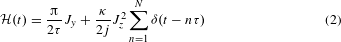where Jy and Jz are angular momentum operators. The sum over delta functions implies N applications of Jz2, each at integer time steps. The angular momentum operators can be expressed in terms of the constituent spin-1/2 Pauli operators, for example, Jz = (/2)∑ iσz(i). Setting τ = 1, the first term in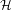causes each spin to rotate around the y-axis by an angle π/2. The second term couples every spin to every other spin with strength κ/2j. This can be seen by expanding Jz2 in terms of z operators, where terms such as σz(1)σz(2) and all other combinations appear.

The classical dynamics, being simple to visualize and interpret, can provide valuable intuition for studying the quantum limit. The classical limit of this model occurs when j is very large and quantization effects become negligible. In this limit, the system behaves like a classical spinning top, with dynamics which are known to be chaotic14,15,16,17. The parameter κ sets the chaoticity and takes the dynamics from regular to chaotic as κ increases; at intermediate values, the system exhibits a rich mixture of both regular and chaotic motion.

Experimentally realizing this model requires a high degree of control over both local terms and interactions in a multi-qubit Hamiltonian. This led to the design of a three-qubit ring of planar transmons with tunable inter-qubit coupling (see Supplementary Information)19,20,21. The rotations around the y-axis (Jy) are performed using shaped microwave pulses that are resonant with the qubit transition. The simultaneous and symmetric three-qubit interaction (Jz2) is turned on and off using a tunable coupling circuit controlled by three separate square pulses. The qubit–qubit interaction energy g and the duration of the interaction pulses T set κ through the relation κ = 3gT/. We measure the strength of the interaction energy κ by determining the time it takes for an excitation to swap between the qubits (see Supplementary Information).

The periodic nature ofallows us to write down the unitary evolution over one cycle as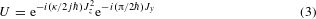shown schematically in Fig. 1a. We begin by initializing each qubit in the state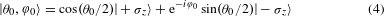where θ0 and φ0 are angles describing the orientation of the single-qubit states. This state is known as a spin coherent state and is the most classical spin state in the sense of minimum uncertainty and zero entanglement. After preparing the initial state, we rotate each qubit around the y-axis by an angle π/2. Next, we allow all of the qubits to interact with one another for a duration which sets the value of κ. We repeat these two steps N times and then tomographically reconstruct the resulting density matrix22. For details regarding the pulse sequence, see Supplementary Information.Figure 1: Pulse sequence and the resulting quantum dynamics.

We visualize the evolution of the system by depicting the single-qubit state as a vector inside of a Bloch sphere, shown in Fig. 1b. Each Bloch vector is constructed by measuring the expectation values of the x, y and z Pauli operators after evolving the system according to equation (3). As the dynamics are symmetric under qubit exchange, the qubits undergo nominally identical evolution and we plot the average behaviour (see Supplementary Information). The chosen initial state is shown in red, with the Bloch vector after subsequent steps shown in blue. After each step, there are two qualitative changes: a rotation and a change in the length. The orientation is analogous to the orientation of the classical spin. The change in length, however, describes entanglement among the qubits.

Entanglement can be characterized using the entanglement entropy S,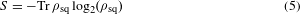where ρsq is the density matrix of a single qubit. Writing the trace as a sum reproduces the familiar definition of entropy −∑ pi log(pi), where pi is the probability of being in the ith microstate. If the qubit is in a pure state, then the single-qubit state is completely known and the entropy is zero. However, if the qubits are entangled with one another, then ρsq is a statistical mixture of states and the entropy is non-zero.

In Fig. 2a, we show the entanglement entropy between a single qubit and the rest of the qubits at several instances in time. The entanglement entropy is measured by performing state tomography on the individual qubits22 and then directly applying equation (5) to the reconstructed density matrix. In each panel, we prepare various initial states |θ0, φ0〉, evolve the system for N steps and plot the entanglement entropy; different panels correspond to different N. Initial states prepared close to the y-axis have low entropy (red), which remains low as the system evolves. States prepared farther away from the y-axis gain higher entropy (blue) given sufficient time. We perform the same set of experiments for stronger interaction, κ = 2.5, shown in Fig. 2b. At stronger interactions, the entropy increases rapidly and regions of low entropy are no longer isolated to near the y-axis.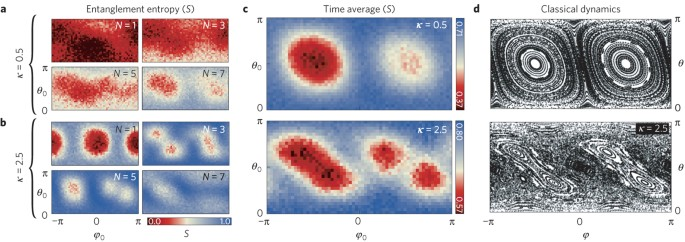Figure 2: Entanglement entropy and classical chaos.

In Fig. 2a, b, we see that the entropy fluctuates over time. In small quantum systems, there are fluctuations or revivals that vanish when the system size is taken to infinity (known as the thermodynamic limit). For finite systems, averaging the entropy over time is commonly used to estimate the equilibrium value approached by larger systems. In Fig. 2c, we show the entanglement entropy averaged over time (N) for both values of interaction strength κ. The corresponding classical dynamics are shown in Fig. 2d.

We find a striking resemblance between the average entanglement in the quantum system and chaotic dynamics in the classical limit. The regions of classical phase space where the dynamics are chaotic correspond to high entropy (blue) in the quantum system; regions that are classically regular correspond to low entropy (red), including bifurcation at large κ. The results shown in Fig. 2b have been predicted by recent theoretical works23,24. The connection between chaos and entanglement has been of theoretical interest for a considerable time25,26,27. However, these studies focused on very large systems near the border of quantum and classical physics28,29. Here, we show that the results hold deep in the quantum limit. It is interesting to note that chaos and entanglement are each exclusive to their respective classical and quantum domains, and any connection is surprising. The correspondence is even more unexpected given that our system is so far from the classical limit6,30.

In Fig. 2b, the entanglement entropy in the blue regions approaches 0.8, close to the maximum attainable value of 1.0 for a single qubit. The value of 0.8 is very close to the value one would obtain by uniformly averaging over all states, 0.73 (ref. 31). In equation (2), the Hamiltonian depends on time and, as a result, energy is not conserved. Therefore, statistical mechanics would predict the values of observables using an ensemble with maximum entropy or, equivalently, an infinite temperature ensemble. The observed density matrix approaching maximum entropy suggests that even small quantum systems undergoing unitary dynamics can appear to thermalize3,32,33.

In the Supplementary Information, we numerically compute the evolution for larger systems and show that fluctuations decrease with increasing system size, as expected for finite-size systems approaching thermal equilibrium. Additionally, we compute the behaviour at larger values of κ and show that all initial states obtain near-maximal entropy, as opposed to the mixed phase space shown in Fig. 2. This further supports the idea that what we see in the experiment is the onset of thermalization in a small quantum system.

The observed single-qubit entropy can originate from two sources: entanglement with the other qubits and entanglement with the environment (decoherence). In Fig. 3, we show that the contrast between high- and low-entropy results from entanglement among the qubits, confirming our assumption that the system is well isolated. To distinguish these two effects, we measure the three-qubit density matrix. Using these measurements, we compute the expectation values of all combinations of Pauli operators. The first nine columns in Fig. 3 contain operators on only a single qubit, and thus provide information about local properties. The remaining columns contain products of two- and three-qubit operators, and describe correlations between the qubits.Figure 3: Multi-qubit entanglement.

In the top panel, we consider an initial state whose entropy has increased by the least amount (most red), shown inset. After ten time steps, we see that each qubit is oriented along the y-axis, as indicated by the first three peaks. The qubits pointing along the same direction lead to classical correlations, as indicated by the remaining peaks among the two- and three-qubit correlations. In the lower panel, we consider an initial state whose entropy has increased by the largest amount (most blue). In addition to the qubit orientations and classical correlations, we also find many significant peaks among the multi-qubit correlations. These non-classical correlations are clear signatures of entanglement among the qubits. Additionally, we find that the three-qubit state purity, a measure of decoherence, is equal for both of these states, showing that the contrast between high and low entropy is entirely the result of inter-qubit entanglement (see Supplementary Information). This finding is in contrast to previous studies which found that, for initial states with high entropy, the system displayed a hypersensitivity to perturbations, such as environmental decoherence17.

The advantage of studying statistical mechanics in a small quantum system is that we can directly check for ergodic motion in the three-qubit dynamics. Using measurements of the full multi-qubit density matrix, we investigate the connection between ergodic dynamics in the full system and entropy production in subsystems. Note that the full system is ideally in a pure state whose entropy is zero and stays zero as the system evolves—this is in stark contrast to subsystems which gain entropy over time through entanglement. While the full system cannot thermalize in the sense of reaching maximum entropy, it can undergo ergodic motion (time averages being equal to state-space averages). In statistical mechanics, a uniform average over states is given by the microcanonical ensemble. In Fig. 4, we plot the overlap of the time-averaged density matrix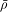with a microcanonical ensemble ρmc, given by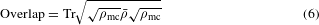Here, ρmc is an 8-by-8 density matrix which attributes equal probability to all of the accessible states. The overlap of these two distributions approaching 1.0 would imply that time averages are equivalent to state-space averages for all measurable quantities.Figure 4: Ergodic dynamics.

We choose three different initial states: two are chosen from regions where subsystems had high entropy (blue and green) and one from a region that had low entropy (red). After just three steps, initial states where subsystems had high entropy approach a microcanonical ensemble to within 94%. Numerical simulations indicate that ideally the overlap plateaus at 98%—deviations from this ideal behaviour are primarily due to decoherence. We find that initial states where subsystems had low entropy fail to approach a microcanonical ensemble. The strong overlap between time averages and state-space averages demonstrates that the three-qubit dynamics are ergodic and further supports the statistical mechanics framework for understanding the entropy production in single qubits.

Previous experiments have investigated the signatures of classical chaos in quantum systems17,34. Here, using our ability to generate arbitrary product states, we establish a clear signature across the entire phase space. Our unique measurement capabilities allow us to go beyond previous works by directly connecting our observations to entanglement among the qubits, as opposed to environmental decoherence. Together, these tools allow us to demonstrate ergodic dynamics and show that superconducting qubits can be used to study fundamental concepts in statistical mechanics.

It is interesting to know the generality of our results, as they could provide a generic framework for studying quantum dynamics. Numerical results suggest that ergodic behaviour breaks down only when the evolution is highly constrained by conservation laws; such systems are referred to as integrable and represent models that are fine tuned and consequently rare3. Our choice of Hamiltonian was motivated by the lack of conserved quantities, where only the total spin is conserved—not even energy is conserved. We believe that our simple and clear descriptions of thermalization merely lay the foundation upon which many fundamental questions in non-equilibrium thermodynamics can be experimentally investigated.

### Data availability.

The data that support the plots within this paper and other findings of this study are available from the corresponding author upon request.

## References

1. 1

Deutsch, J. M. Quantum statistical mechanics in a closed system. Phys. Rev. A 43, 2046–2049 (1991).

2. 2

Srednicki, M. Chaos and quantum thermalization. Phys. Rev. E 50, 888–901 (1994).

3. 3

Rigol, M., Dunjko, V. & Olshanii, M. Thermalization and its mechanism for generic isolated quantum systems. Nature 452, 854–858 (2008).

4. 4

Polkovnikov, A., Sengupta, K., Silva, A. & Vengalattore, M. Colloquium: nonequilibrium dynamics of closed interacting quantum systems. Rev. Mod. Phys. 83, 863–883 (2011).

5. 5

Ott, E. Chaos in Dynamical Systems (Cambridge Univ. Press, 2002).

6. 6

Gutzwiller, M. Chaos in Classical and Quantum Mechanics Vol. 1 (Springer Science and Business Media, 1990).

7. 7

Kinoshita, T., Wenger, T. & Weiss, D. A quantum Newton’s cradle. Nature 440, 900–903 (2006).

8. 8

Klaers, J., Vewinger, F. & Weitz, M. Thermalization of a two-dimensional photonic gas in a white wall photon box. Nature Phys. 6, 512–515 (2010).

9. 9

Cheneau, M. et al. Light-cone-like spreading of correlations in a quantum many-body system. Nature 481, 484–487 (2012).

10. 10

Trotzky, S. et al. Probing the relaxation towards equilibrium in an isolated strongly correlated one-dimensional Bose gas. Nature Phys. 8, 325–330 (2012).

11. 11

Langen, T., Geiger, R., Kuhnert, M., Rauer, B. & Schmiedmayer, J. Local emergence of thermal correlations in an isolated quantum many-body system. Nature Phys. 9, 640–643 (2013).

12. 12

Richerme, P. et al. Non-local propagation of correlations in quantum systems with long-range interactions. Nature 511, 198–201 (2014).

13. 13

Schreiber, M. et al. Observation of many-body localization of interacting fermions in a quasi-random optical lattice. Science 349, 842–845 (2015).

14. 14

Wang, X., Ghose, S., Sanders, B. C. & Hu, B. Entanglement as a signature of quantum chaos. Phys. Rev. E 70, 016217 (2004).

15. 15

Ghose, S., Stock, R., Jessen, P., Lal, R. & Silberfarb, A. Chaos, entanglement, and decoherence in the quantum kicked top. Phys. Rev. A 78, 042318 (2008).

16. 16

Lombardi, M. & Matzkin, A. Entanglement and chaos in the kicked top. Phys. Rev. E 83, 016207 (2011).

17. 17

Chaudhury, S., Smith, A., Anderson, B., Ghose, S. & Jessen, P. Quantum signatures of chaos in a kicked top. Nature 461, 768–771 (2009).

18. 18

Haake, F., Kuś, M. & Scharf, R. Classical and quantum chaos for a kicked top. Z. Phys. B 65, 381–395 (1987).

19. 19

Barends, R. et al. Coherent Josephson qubit suitable for scalable quantum integrated circuits. Phys. Rev. Lett. 111, 080502 (2013).

20. 20

Chen, Y. et al. Qubit architecture with high coherence and fast tunable coupling. Phys. Rev. Lett. 113, 220502 (2014).

21. 21

Geller, M. et al. Tunable coupler for superconducting Xmon qubits: perturbative nonlinear model. Phys. Rev. A 92, 012320 (2015).

22. 22

Neeley, M. et al. Generation of three-qubit entangled states using superconducting phase qubits. Nature 467, 570–573 (2010).

23. 23

Khripkov, C., Cohen, D. & Vardi, A. Coherence dynamics of kicked Bose–Hubbard dimers: interferometric signatures of chaos. Phys. Rev. E 87, 012910 (2013).

24. 24

Madhok, V., Gupta, V., Hamel, A. & Ghose, S. Signatures of chaos in the dynamics of quantum discord. Phys. Rev. E 91, 032906 (2015).

25. 25

Bandyopadhyay, J. & Lakshminarayan, A. Testing statistical bounds on entanglement using quantum chaos. Phys. Rev. Lett. 89, 060402 (2002).

26. 26

Lakshminarayan, A. Entangling power of quantized chaotic systems. Phys. Rev. E 64, 036207 (2001).

27. 27

Miller, P. & Sarkar, S. Signatures of chaos in the entanglement of two coupled quantum kicked tops. Phys. Rev. E 60, 1542–1550 (1999).

28. 28

Boukobza, E., Moore, M., Cohen, D. & Vardi, A. Nonlinear phase-dynamics in a driven bosonic Josephson junction. Phys. Rev. E 104, 240402 (2010).

29. 29

Boukobza, E., Chuchem, M., Cohen, D. & Vardi, A. Phase-diffusion dynamics in weakly coupled Bose–Einstein condensates. Phys. Rev. Lett. 102, 180403 (2009).

30. 30

Berry, M. The Bakerian Lecture, 1987: Quantum chaology. Proc. R. Soc. Lond. A 413, 183–198 (1987).

31. 31

Page, D. Average entropy of a subsystem. Phys. Rev. Lett. 71, 1291–1294 (1993).

32. 32

Santos, L., Polkovnikov, A. & Rigol, M. Weak and strong typicality in quantum systems. Phys. Rev. E 86, 010102 (2012).

33. 33

Polkovnikov, A. Microscopic diagonal entropy and its connection to basic thermodynamic relations. Ann. Phys. 326, 486–499 (2011).

34. 34

Lemos, G., Gomes, R., Walborn, S., Ribeiro, P. & Toscano, F. Experimental observation of quantum chaos in a beam of light. Nature Commun. 3, 1211 (2012).

Download references

## Acknowledgements

We acknowledge discussions with M. Fisher, P. Jessen, V. Madhok, C. Nayak, A. Pattanayak, T. Prosen, A. Rahmani and D. Weld. This work was supported by the NSF under grants DMR-0907039 and DMR-1029764, the AFOSR under FA9550-10-1- 0110, and the ODNI, IARPA, through ARO grant W911NF-10-1-0334. Devices were made at the UCSB Nanofab Facility, part of the NSF-funded NNIN, and the NanoStructures Cleanroom Facility.

## Author information

Authors

### Contributions

C.N., P.R. and Y.C. designed and fabricated the sample and co-wrote the manuscript. C.N., P.R. and M.F. designed the experiment. C.N. performed the experiment and analysed the data. M.K. and A.P. provided theoretical assistance. All members of the UCSB team contributed to the experimental set-up and to the manuscript preparation.

### Corresponding author

Correspondence to C. Neill.

## Ethics declarations

### Competing interests

The authors declare no competing financial interests.

## Supplementary information

### Supplementary information

Supplementary information (PDF 5660 kb)

## Rights and permissions

Reprints and Permissions

## Further reading

• ### Fermion-induced dynamical critical point

• Shuai Yin
•  & Shao-Kai Jian

Physical Review B (2021)

• ### Emergence and control of complex behaviors in driven systems of interacting qubits with dissipation

• A. V. Andreev
• , A. G. Balanov
• , T. M. Fromhold
• , M. T. Greenaway
• , A. E. Hramov
• , W. Li
• , V. V. Makarov
•  & A. M. Zagoskin

npj Quantum Information (2021)

• ### Quantum metrology via chaos in a driven Bose-Josephson system

• Wenjie Liu
• , Min Zhuang
• , Bo Zhu
• , Jiahao Huang
•  & Chaohong Lee

Physical Review A (2021)

• ### General method to sample systems in the microcanonical ensemble using Monte Carlo simulations

• G. Palma
•  & A. Riveros

The European Physical Journal B (2021)

• ### Entanglement Dynamics of Random GUE Hamiltonians

• Daniel Chernowitz
•  & Vladimir Gritsev

SciPost Physics (2021)

## Search

### Quick linksSign up for the Nature Briefing newsletter — what matters in science, free to your inbox daily.

Get the most important science stories of the day, free in your inbox. Sign up for Nature Briefing